Freezing Constraints and Variables
After solving at each priority, the RiverWare Optimization solution guarantees that later priorities will not degrade the objective from the current priority by locking in or freezing portions of the solution. See Preemptive Goal Programming for conceptual examples of this. For numeric stability reasons, RiverWare does not technically freeze the objective function itself but rather freezes constraints and variables. These are constraints that are forced to their limits by the objective and variables that are forced to their upper or lower bounds. Freezing the appropriate constraints and variables guarantees that the objective will not be degraded by lower priorities. Violations of soft constraints always results in freezing that removes degrees of freedom from the solution. If there are no violations, freezing usually reduces the size of the feasible polyhedron without removing degrees of freedom.This section provides details about the freezing of constraints and variables.
Information about frozen constraints and objectives is helpful in Optimization solution analysis. Constraints that get frozen at the priority that they are introduced, which are typically soft constraints that cannot be fully satisfied, are constraints that are driving the solution at that priority. Constraints that were introduced at an earlier priority and get frozen at a lower priority are limiting the objective function at the lower priority. In the case of unsatisfied soft constraints at a given priority, the frozen constraints that were introduced at an earlier priority are preventing the constraints from being fully satisfied. See Priority-oriented Optimization Solution Analysis Tool for details about frozen constraint information in the Optimization Solution Analysis Tool.
Freezing always occurs automatically after each iteration of a Repeated Maximin derived objective. This is necessary given the nature of the solution. A second Repeated Maximin iteration at a given priority only makes sense when constraints and variables are frozen after the first iteration. Otherwise it would simply be solving the same problem over and over. For all other objective types (Maximize, Minimize, Summation, Single Maximin), an explicit Freeze statement must be added to the goal after the objective statement. See Freeze for details about how to apply a Freeze statement to goals. A common mistake is forgetting to add the Freeze statement after these objectives. If the Freeze statement is omitted, the solution will look the same as if the goal was never included in the optimization policy. The reason for this design is to retain the ability to have test objectives that provide information for a subsequent goal without using it to freeze the solution. This approach is not common, but when needed, it is very useful.
Freezing Constraints
Assume that at priority 1 a reservoir has a Minimum Storage constraint for every timestep.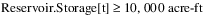At priority 2, the reservoir has a Minimum Outflow constraint.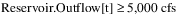Assume that, given the initial Storage and Inflow over the first three timesteps (t1, t2, t3), it is not possible to meet both the Minimum Outflow and the Minimum Storage. The Minimum Storage is at a higher priority, so the solution would satisfy those constraints, and it would get the Outflow as close to the Minimum of 5,000 cfs as possible. In doing so, it would take the Storage down to the minimum by the third timestep. Using as much water as is available from the reservoir is the means by which it would get as close to the Minimum Outflow as possible. In other words, the Minimum Outflow constraint at priority 2 forces the Minimum Storage from priority 1 to be tight on t3, and the Minimum Storage constraint on t3 is limiting the objective for priority 2.
For all priorities below priority 2, we already know that the Storage at t3 must be at the Minimum Storage in order to prevent degrading the priority 2 objective to maximize the satisfaction of the Minimum Outflow constraint. So RiverWare freezes the Minimum Storage constraint on t3 by changing it to an “equal-to” constraint.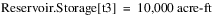This would remove a degree of freedom from the solution for all subsequent priorities. The Storage on t1 and t2 is still constrained to be greater-than-or-equal-to 10,000 acre-ft, but at this point they have not been forced to be equal to 10,000 acre-ft, so they are not frozen here.
Technically, the constraint would be frozen in its canonical form including the satisfaction variable; see Satisfaction Variables in Soft Constraints for details. Assume that the initial lower bound on Reservoir Storage before priority 1 was 0 acre-ft, then this would look like the following: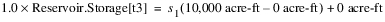where 1.0 is the coefficient on the Reservoir Storage variable, s1 is the satisfaction variable from priority 1, which would have been frozen at a value of 1.0 when solving at priority 1, and 0 acre-ft was the previous bound on Reservoir Storage.
Note:  Technically, all evaluation is done internally in RiverWare Optimization units, which are typically scaled SI units, not in user display units as shown here.
The Minimum Outflow constraints would also get frozen at priority 2 but with a satisfaction variable value less than 1. Assume that the previous bound on Outflow was 0 cfs, and the priority 2 solution was only able to get the outflow to 4,000 cfs on all three timesteps. The satisfaction would be 0.8 (80% from the old bound of 0 cfs to the new constraint value of 5,000 cfs). The Minimum Outflow constraint would be frozen as follows: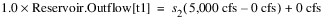where s2 is the satisfaction variable for priority 2 and will be frozen at a value of 0.8. The Minimum Outflow constraints at t2 and t3 would be frozen in a similar manner.
RiverWare determines which constraints to freeze by evaluating the dual price for each constraint. The dual price represents the unit improvement in the objective function that would result from a unit change in the constraint right-hand-side value while holding all else constant. If a constraint’s dual price is greater than 0, it is an indication that the constraint is limiting the objective (i.e. the constraint is binding) so that constraint gets frozen. For example, assume that reducing the Minimum Storage limit on t3 from 10,000 acre-ft to 9,999 acre-ft would allow an Outflow of 4,000.2 cfs on all three timesteps. This would improve the objective value from 80% to 80.004%. The dual price of the Minimum Storage constraint on t3 would be 0.004%/acre-ft. This is greater than zero, so the Minimum Storage constraint on t3 gets frozen. If the Minimum Storage value on t1 or t2 were relaxed, the objective would still be limited by the Minimum Storage on t3. The the objective value would not be improved by changing the t1 or t2 constraint value. Thus the dual price for these constraints is zero, and the constraints do not get frozen. Internally this evaluation is always carried out in RiverWare Optimization units, and the dual price must actually be greater than a freezing tolerance of 10-6 in RiverWare Optimization units in order to freeze the constraint.
When looking at the Optimization Solution Analysis Tool for priority 2, you would see the Minimum Outflow constraints as constraints from the current priority that were frozen. See Priority-oriented Optimization Solution Analysis Tool for details. These constraints were driving the solution at priority 2. You would also see the Minimum Storage constraint from t3 as a constraint that was introduced earlier that was frozen at priority 2, indicating that it limited the satisfaction of the priority 2 Minimum Outflow constraints.
Freezing Variables
Variables that are binding on the solution get frozen as well. This occurs when variables are forced to their upper or lower bound by the objective. (For variables that correspond to slots, the upper and lower bounds are set in their slot configuration by the user. See Variable Bounds for information about setting the required bounds on variables. For variables that are added to the problem automatically by RiverWare and do not correspond to slots, RiverWare sets the bounds automatically. One example of the latter is the satisfaction variable for a soft constraint.)
For example, Regulated Spill typically has a lower bound of zero. Assume that there are no policy constraints that require Regulated Spill to be greater-than-or-equal-to a non-zero value. An objective to maximize Power generation will often drive Regulated Spill to zero in order to use as much water as possible for generation. The variable will get frozen at its lower bound of zero.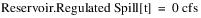Another common case in which variables are frozen is the freezing of satisfaction variables for soft constraints. Satisfaction variables automatically have upper and lower bounds of 0 and 1. For example, assume that the goal at priority i contains a Repeated Maximin derived objective. RiverWare will add a new satisfaction variable, si, to the problem.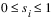If the solution is able to fully satisfy all of the constraints in goal i, then it will freeze the satisfaction constraint at its upper bound of 1.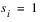Similar to the use of dual prices for freezing constraints, reduced costs are used in the freezing of variables. RiverWare freezes all variables with a reduced cost greater than zero. The reduced cost represents the unit decrease in the objective value for a unit change in the bound on the variable (unit increase for a lower bound or unit decrease for an upper bound). In the Regulated Spill example, increasing the lower bound on Regulated Spill to be greater than zero would decrease the amount of power that could be generated. Some water would have to be used for spill rather than generation. Thus increasing the lower bound would reduce the objective value, so Regulated Spill would have a non-zero reduced cost. If the upper bound on the satisfaction variable in the second example were less than one, it would reduce the objective to maximize satisfaction, so the reduced cost on the satisfaction variable is non-zero.
In this case that the satisfaction variable is frozen at a value of 1, it is as if the objective is frozen directly. This is not the case in general, however. The satisfaction variable only gets frozen if it is at its bound. (Typically this is when the satisfaction variable is at its upper bound of 1, but it can also occur if a satisfaction variable on an individual constraint in a Summation derived objective is at its lower bound of zero.) If the satisfaction is somewhere between 0 and 1, the satisfaction will not get frozen directly. Freezing all constraints with a non-zero dual price and all variables with a non-zero reduced cost has the equivalent effect of freezing the objective value but performs much better in terms of numeric stability.
Typically information about frozen variables is not as useful as information about frozen constraints when trying to understand the Optimization solution. Often this is due to the fact that when a variable gets frozen at a bound, this is an obvious limit that the user considers intuitively. Thus it does not tend to provide the user with more information than they already had. In some cases, however, identifying unexpected frozen variables can assist in debugging an Optimization model, particularly if incorrect upper or lower bounds were set on a variable unintentionally.
Revised: 08/02/2021